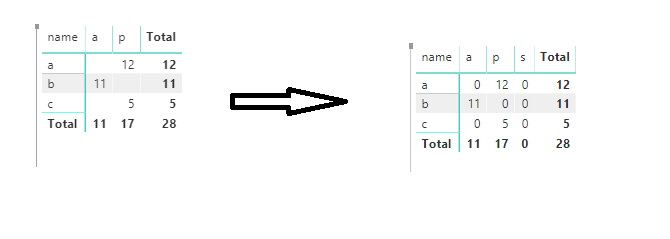cancel
Showing results for
Did you mean:Helper II

## Matrix Visual - Show Blank Values as Zero

Hello,

I'm using a matrix visual with many columns.  I would like to show blank values as zero.  In Excel, there is a simple option checkbox for this, but I can't seem to find any equivalent in PowerBI.  I know one solution would be along the lines of switching to a table visual, creating a separate measure for each column and adding zero at the end of the measure, but I would really prefer to avoid having to do this given the number of columns.  Does anyone know of a simpler solution?

Thanks!

1 ACCEPTED SOLUTIONCommunity Support

Hi mstone3,

If you want to show blank value  as zero like belowYou could use expression like below

`Column= marix[amoaunt]+0`

If you want to fill blank area with zero in matrix like belowBecause the records don’t exist in dataset, so it will show blank. So you need to create a related table like belowUse this filed in Matrix, then create measure like below

`Measure 7 = SUM(marix[amoaunt])+0`Best Regards,

Zoe Zhi

If this post helps, then please consider Accept it as the solution to help the other members find it more quickly.Community Support

Hi mstone3,

If you want to show blank value  as zero like belowYou could use expression like below

`Column= marix[amoaunt]+0`

If you want to fill blank area with zero in matrix like belowBecause the records don’t exist in dataset, so it will show blank. So you need to create a related table like belowUse this filed in Matrix, then create measure like below

`Measure 7 = SUM(marix[amoaunt])+0`Best Regards,

Zoe Zhi

If this post helps, then please consider Accept it as the solution to help the other members find it more quickly.